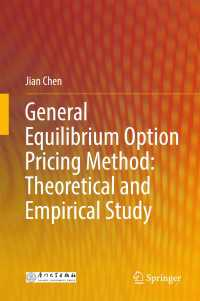## 電子書籍詳細General Equilibrium Option Pricing Method: Theoretical and Empirical Study . 1st ed. 2018

Chen, Jian

Springer 2018/04
XI, 164 p. 31 illus., 10 illus. in color.

ISBN: 9789811074271
eISBN: 9789811074288
KNPID: EY00239265

ファイルフォーマット:
ファイルサイズ:
デバイス:ご購入を希望される方は、下のリンクをクリックしてください。

### Full Description

This book mainly addresses the general equilibrium asset pricing method in two aspects: option pricing and variance risk premium. First, volatility smile and smirk is the famous puzzle in option pricing. Different from no arbitrage method, this book applies the general equilibrium approach in explaining the puzzle. In the presence of jump, investors impose more weights on the jump risk than the volatility risk, and as a result, investors require more jump risk premium which generates a pronounced volatility smirk. Second, based on the general equilibrium framework, this book proposes variance risk premium and empirically tests its predictive power for international stock market returns.

### Table of Contents

Chapter1.Introduction.- Chapter2.General Equilibrium Option Pricing Models.- Chapter3.Simulation Comparison.- Chapter4.Empirical Comparison.- Chapter5.Fanning Preference and Option Pricing.- Chapter6.Jump Size Distribution and Option Pricing.- Chapter7.Risk Aversion Estimated From Variance Risk Premium.-Chapter8.Predictability of Variance Risk Premium: Hong Kong Evidence.- Chapter9.Predictability of Variance Risk Premium:Other International Evidence.- Chapter10.Predictability of Variance Risk Premium:A Comparison Study.- Chapter11.Conclusions.